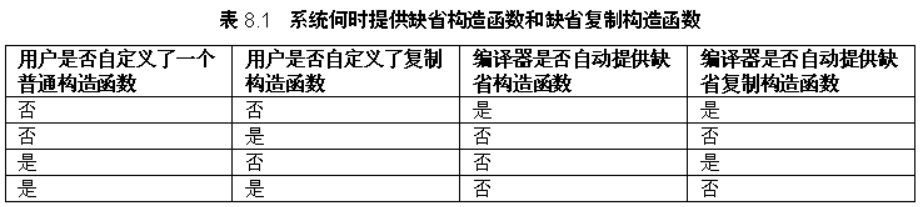# c++之拷贝构造函数详解

C++中经常使用一个常量或变量初始化另一个变量，例如：

double x＝5.0；

double y＝x;

point pt1(2,3);

point pt2=pt1;

point pt2( pt1);

point:: point (const point &);

注意：当我们自己定义了有参构造函数时，系统不再提供默认构造函数。这是容易忽略的一点。

point pt1(3,4); //构造函数 p

oint pt2(pt1); //复制构造函数

point pt3 = pt1;//复制构造函数

#include <iostream>
using namespace std;
class point
{
private:
int xPos;
int yPos;
public:
point(int x = 0, int y = 0)
{
cout << "调用构造函数" << endl;
xPos = x;
yPos = y;
}
point(const point & pt)//复制构造函数的定义及实现
{
cout << "调用复制构造函数" << endl;
xPos = pt.xPos;
yPos = pt.yPos;
}
void print()
{
cout << "xPos: " << xPos << ",yPos: " << yPos << endl;
}
};
#include "point.h"

int main()
{
point pt1(3, 4);
pt1.print();

point pt2 = pt1; //等价于point pt2(pt1)，调用复制构造函数
pt2.print();

point pt3;
pt3.print();

point pt4(pt3); //等价于point pt4＝pt3，调用复制构造函数
pt4.print();

//	pt2 = pt1;		//调用默认的赋值运算符重载函数
//	pt2.print();
return 0;
}

#include <iostream>
using namespace std;

class CPoint
{
private:
int x;
int y;

public:
//缺省构造函数,如果定义类时未指定任何构造函数，
//系统将自动生成不带参数的缺省构造函数
CPoint()
{
cout << "默认构造函数 " << this << " " << endl;
x = 0;
y = 0;
}

//带一个参数的可用于类型转换的构造函数
CPoint(int ix)
{
cout << "1参数构造函数 " << this << " " << endl;
x = ix;
y = 0;
}

//带参数的构造函数
CPoint(int ix, int iy)
{
cout << "2参数构造函数 " << this << " " << endl;
x = ix;
y = iy;
}

//拷贝构造函数,如果此函数不定义，系统将生成缺省拷贝构造函数功能,
//缺省拷贝构造函数的行为是：用传入的对象参数的成员初始化正要建立的对象的相应成员
CPoint(const CPoint &cp)
{
cout << "拷贝构造函数 " << this << " " << endl;
x = cp.x;
y = cp.y;
}

CPoint &operator=(const CPoint &cp)
{
cout << "赋值重载函数 " << this << " " << endl;
if (this != &cp)
{
x = cp.x;
y = cp.y;
}
return (*this);
}

//析构函数，一个类中只能有一个析构函数,如果用户没有定义析构函数，
//系统会自动未类生成一个缺省的析构函数
~CPoint()
{
cout << "析构函数 " << this << " " << endl;
}
};

void fun1(CPoint pt)
{
}

CPoint fun2()
{
CPoint a;
return a;
}

CPoint fun(CPoint a)
{
return a;
}

int main(int argc, char* argv[])
{
//第1类
//	CPoint pt1 = CPoint();
//当有析构函数的时候，CPoint()不会生成调用构造函数生成临时的匿名对象。
//当没有析构函数的时候，CPoint()会生成一个临时的匿名对象，等价于CPoint pt1;这句话只会调用无参构造函数，不会调用拷贝构造函数

//	CPoint pt2 = CPoint(1);
//当有析构函数的时候，CPoint(1)不会生成调用构造函数生成临时的匿名对象。
//当没有析构函数的时候，CPoint()会生成一个临时的匿名对象，等价于CPoint pt(1);这句话只会调用一个参数的构造函数，不会调用拷贝构造函数

//	CPoint pt3 = 1;
//普通数据类型转换为类类型，利用相应的构造函数就可以实现。等价于CPoint pt(1);

//第2类
/*拷贝构造函数与赋值运算符重载函数的区别:
1. 拷贝构造函数是用已经存在的对象的各成员的当前值来创建一个相同的新对象。
在下述3种情况中，系统会自动调用所属类的拷贝构造函数。
1.1  当说明新的类对象的同时，要给它赋值另一个已经存在对象的各成员当前值。
1.2  当对象作为函数的赋值参数而对函数进行调用要进行实参和形参的结合时。
1.3  当函数的返回值是类的对象，在函数调用结束后返回主调函数处的时候。
2. 赋值运算符重载函数要把一个已经存在对象的各成员当前值赋值给另一个已经存在的同类对象
*/
CPoint pt4;			//调用无参构造函数
//	CPoint pt5 = pt4;	//调用拷贝构造函数  属于1.1
//	fun1(pt4);			//调用拷贝构造函数  属于1.2
//	fun2();				//调用拷贝构造函数  属于1.3

//	CPoint pt6 = fun2();//调用无参构造函数，拷贝构造函数，此处如果没有写析构函数，则还会调用一次拷贝构造函数
//因为函数返回会生成一个临时对象，然后再将这个临时对象赋值给pt6，所以多调用一次拷贝构造函数；如果有析构函数
//则不会生成中间的临时变量，所以少一次拷贝构造函数的调用

//还可以通过下面函数验证
//	CPoint pt7 = fun(pt4);
//如果没有析构函数，会调用3次拷贝构造函数

return 0;
}


拷贝构造函数在以下三种情况会自动调用：

当把一个已经存在的对象赋值给另一个新的对象时。

当实参和形参都是对象，进行形参和实参的结合时。

当函数的返回值是对象，函数调用完成返回时。

computer comp2(comp1)

comp2.brand = comp1.brand;

comp2.price = comp1.price;

computer(const computer &cp) //自定义复制构造函数

{

//重新为brand开辟和cp.brand同等大小的动态内存

brand = new char[strlen(cp.brand) + 1];

strcpy(brand, cp.brand); //字符串复制 price = cp.price;

}CTest t0(); //这是函数的声明，不是实例化类

CTest t1; //缺省构造函数

CTest t2(1); //一个参数的构造函数

CTest t3(1, 2); //两个参数的构造函数

CTest t4 = 1; //等价于CTest t4(1); //explicit

CTest t5 = t1; //CTest(t1);

CTest t6 = CTest();//CTest(1);

CTest(1,2);

t6 = CTest(1);

t6 = 1; //首先调用单个参数的构造函数，生成临时 //对象CTest(1), 然后调用赋值运算符函数

t6 = t1; //调用赋值运算符函数 见备注代码。请注意输出的地址值，观察构造函数和析构函数的配对情况。

//为了防止CPoint pt = 2;和CPoint pt2 = pt1;这种隐性转换，可以在相应的构造函数前增加explicit标识符
#include <iostream>
using namespace std;

class CPoint
{
protected:
int x;
int y;

public:
//缺省构造函数,如果定义类时未指定任何构造函数，
//系统将自动生成不带参数的缺省构造函数
CPoint()
{
cout << "默认构造函数 " << this << " ";
x = 0;
y = 0;
}

//带一个参数的可用于类型转换的构造函数
//	explicit	//加上 explicit 可防止 CPoint pt1 = 1; 这种隐性转换
CPoint(int ix)
{
cout << "1参数构造函数 " << this << " ";
x = ix;
y = 0;
}

//带参数的构造函数
CPoint(int ix, int iy)
{
cout << "2参数构造函数 " << this << " ";
x = ix;
y = iy;
}

//拷贝构造函数,如果此函数不定义，系统将生成缺省拷贝构造函数功能,
//缺省拷贝构造函数的行为是：用传入的对象参数的成员初始化正要建立的对象的相应成员
//	explicit	//加上 explicit 可防止 CPoint pt2 = pt1; 这种隐性转换
CPoint(const CPoint &cp)
{
cout << "拷贝构造函数 " << this << " ";
x = cp.x;
y = cp.y;
}

CPoint &operator=(const CPoint &cp)
{
cout << "赋值重载函数 " << this << " ";
if (this != &cp)
{
x = cp.x;
y = cp.y;
}
return (*this);
}

//析构函数，一个类中只能有一个析构函数,如果用户没有定义析构函数，
//系统会自动未类生成一个缺省的析构函数
~CPoint()
{
cout << "析构函数 " << this << " ";
}
};

int main(int argc, char* argv[])
{
CPoint p0();		//这是函数的声明，不是实例化类

cout << endl << "CPoint pt1;\t\t";
CPoint pt1;			//缺省构造函数

cout << endl << "CPoint pt2(1);\t\t";
CPoint pt2(1);		//一个参数的构造函数

cout << endl << "CPoint pt3(1, 2);\t";
CPoint pt3(1, 2);	//两个参数的构造函数

cout << endl << "CPoint pt4 = 1;\t\t";
CPoint pt4 = 1;		//等价于CPoint t4(1);	//explicit

cout << endl << "CPoint pt5 = t1;\t";
CPoint pt5 = pt1;	//CPoint(t1);

cout << endl << "CPoint pt6 = CPoint();\t";
CPoint pt6 = CPoint();	//CPoint(1); CPoint(1,2);

cout << endl << "pt6 = CPoint(1);\t";
pt6 = CPoint(1);

cout << endl << "pt6 = 1;\t\t";
pt6 = 1;			//首先调用单个参数的构造函数，生成临时对象CPoint(1), 然后调用赋值运算符函数

cout << endl << "pt6 = t1;\t\t";
pt6 = pt1;			//调用赋值运算符函数

cout << endl << endl;
return 0;
}


posted @ 2018-06-06 21:41  CTHON  阅读(17248)  评论(0编辑  收藏